permutation

(redirected from Arrangement number)
Also found in: Dictionary, Thesaurus, Medical, Legal.

permutation

1. Maths
a. an ordered arrangement of the numbers, terms, etc., of a set into specified groups
b. a group formed in this way. The number of permutations of n objects taken r at a time is n&! / (n--r)&!
2. a fixed combination for selections of results on football pools

Permutation

the replacement of each of the elements a of a given set by another element ϕ(a) of the same set. Each element of the initial set must be obtained precisely once as a result of the permutation. Thus, a permutation is essentially a one-to-one mapping of a set onto itself. The concept of permutation is applied chiefly to finite sets, and only this case will be considered here.

A permutation is commonly symbolized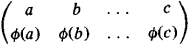Here, each element of the given set is written above the element corresponding to it. Since the properties of a permutation are independent of the nature of the elements a, b, …, c, the latter are usually replaced by the integers 1, 2, …, n. In the upper row, the integers are usually written in their natural order. The permutation then has the form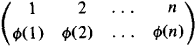or simplywhere ϕ1, ϕ2, …, ϕn are the numbers 1, 2, …, n, possibly in a different order. Thus, the second row of a permutation is an arrangement, ϕ1, ϕ2, …, ϕn of the numbers 1, 2, …, n. There are as many different permutations of n elements as there are arrangements—that is, n! = 1 × 2 × 3 × … × n.

The permutation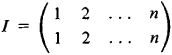which leaves invariant all elements, is called the identity permutation. Every permutation A has an inverse, that is, a permutation that carries ϕi to i. The inverse of A is denoted by A–1. For example, if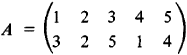then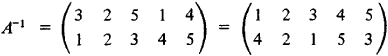The result of the successive application of two permutations A and B is itself a permutation C. If A carries i to ϕi and B carries ϕi to ψi, then C carries i to ψi. C is called the product of A and B; this relationship is written C = AB. For example, if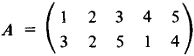andthenMultiplication of permutations is not commutative; that is, in general ABBA. In the above example,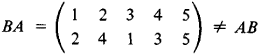It can be easily seen that IA = AI = A, that AA–1 = A–1A = I, and that the associative law A(BC) = (AB)C holds. Thus, all the permutations of n elements form a group, which is called the symmetric group of degree n.

A permutation that interchanges two elements i and j is called a transposition and is denoted by (i, j); for example,Any permutation can be factored into a product of transpositions. When a given permutation is factored into a product of transpositions in different ways, there will be either an even or an odd number of factors. The permutation will accordingly be said to be even or odd; for example, A = (1, 3) (5, 4) (5, 1) is an odd permutation. Define an inversion as an ordered pair of natural numbers such that the first is greater than the second. It turns out that the parity of a permutation can also be determined from the number of inversions in the lower row of the permutation if the numbers in the upper row are arranged in their natural order. The parity of the permutation coincides with the parity of the number of inversions. For example, the lower row of A contains five inversions: (3, 2), (3, 1), (2, 1), (5, 1), and (5, 4). There exist n!/2 even and n!/2 odd permutations of n elements.

A permutation that cyclically permutes a given group of elements while leaving invariant the other elements is called a cycle. The number of permuted elements is called the length of the cycle. For example, A is a cycle of length four: it carries 1 to 3, 3 to 5, 5 to 4, and 4 to 1. This fact is often denoted simply by A = (1, 3, 5, 4). A transposition is a cycle of length two. Any permutation can be factored into a product of disjoint cycles, that is, a product of cycles without common elements. For example,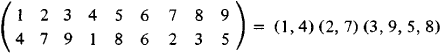REFERENCE

Kurosh, A. G. Kurs vysshei algebry, 10th ed. Moscow-Leningrad, 1971.

permutation

[‚pər·myə′tā·shən]
(mathematics)
A function which rearranges a finite number of symbols; more precisely, a one-to-one function of a finite set onto itself.

permutation

(mathematics)
1. An ordering of a certain number of elements of a given set.

For instance, the permutations of (1,2,3) are (1,2,3) (2,3,1) (3,1,2) (3,2,1) (1,3,2) (2,1,3).

Permutations form one of the canonical examples of a "group" - they can be composed and you can find an inverse permutation that reverses the action of any given permutation.

The number of permutations of r things taken from a set of n is

n P r = n! / (n-r)!

where "n P r" is usually written with n and r as subscripts and n! is the factorial of n.

What the football pools call a "permutation" is not a permutation but a combination - the order does not matter.

2. A bijection for which the domain and range are the same set and so

f(f'(x)) = f'(f(x)) = x.

permutation

One possible combination of items out of a larger set of items. For example, with the set of numbers 1, 2 and 3, there are six possible permutations: 12, 21, 13, 31, 23 and 32.
Site: Follow: Share:
Open / Close